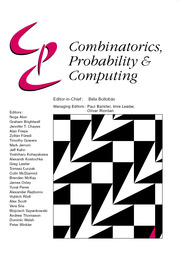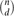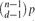HomeCombinatorics, Probability and Computing

# The Asymptotic Number of Connected d-Uniform Hypergraphs†

Published online by Cambridge University Press:  13 February 2014

Corresponding

## Abstract

For d ≥ 2, let Hd(n,p) denote a random d-uniform hypergraph with n vertices in which each of the$\binom{n}{d}$ possible edges is present with probability p=p(n) independently, and let Hd(n,m) denote a uniformly distributed d-uniform hypergraph with n vertices and m edges. Let either H=Hd(n,m) or H=Hd(n,p), where m/n and$\binom{n-1}{d-1}p$ need to be bounded away from (d−1)−1 and 0 respectively. We determine the asymptotic probability that H is connected. This yields the asymptotic number of connected d-uniform hypergraphs with given numbers of vertices and edges. We also derive a local limit theorem for the number of edges in Hd(n,p), conditioned on Hd(n,p) being connected.

## Keywords

Type
Paper
Information
Combinatorics, Probability and Computing , May 2014 , pp. 367 - 385

## Access options

Get access to the full version of this content by using one of the access options below.

## Footnotes

An extended abstract version of this work appeared in the proceedings of RANDOM 2007, Vol. 4627 of Lecture Notes in Computer Science, Springer, pp. 341–352.

## References

Andriamampianina, T. and Ravelomanana, V. (2005) Enumeration of connected uniform hypergraphs. In Proc. FPSAC 2005.Google Scholar
Behrisch, M., Coja-Oghlan, A. and Kang, M. (2007) Local limit theorems for the giant component of random hypergraphs. In Proc. RANDOM 2007, Vol. 4627 of Lecture Notes in Computer Science, Springer, pp. 341352.Google Scholar
Behrisch, M., Coja-Oghlan, A. and Kang, M. (2010) The order of the giant component of random hypergraphs. Random Struct. Alg. 36 149184.Google Scholar
Behrisch, M., Coja-Oghlan, A. and Kang, M. (2014) Local limit theorems for the giant component of random hypergraphs. Combin. Probab. Comput. doi:10.1017/S0963548314000017CrossRefGoogle Scholar
Bender, E. A., Canfield, E. R. and McKay, B. D. (1990) The asymptotic number of labeled connected graphs with a given number of vertices and edges. Random Struct. Alg. 1 127169.CrossRefGoogle Scholar
Bender, E. A., Canfield, E. R. and McKay, B. D. (1992) Asymptotic properties of labeled connected graphs. Random Struct. Alg. 3 183202.CrossRefGoogle Scholar
Bollobás, B. (2001) Random Graphs, second edition, Cambridge University Press.CrossRefGoogle Scholar
Coja-Oghlan, A., Moore, C. and Sanwalani, V. (2007) Counting connected graphs and hypergraphs via the probabilistic method. Random Struct. Alg. 31 288329.CrossRefGoogle Scholar
Erdős, P. and Rényi, A. (1959) On random graphs I. Publicationes Math. Debrecen 5 290297.Google Scholar
Erdős, P. and Rényi, A. (1960) On the evolution of random graphs. Publ. Math. Inst. Hungar. Acad. Sci. 5 1761.Google Scholar
van der Hofstad, R. and Spencer, J. (2006) Counting connected graphs asymptotically. European J. Combin. 27 12941320.CrossRefGoogle Scholar
Janson, S., Łuczak, T. and Ruciński, A. (2000) Random Graphs, Wiley.CrossRefGoogle Scholar
Karoński, M. and Łuczak, T. (1997) The number of connected sparsely edged uniform hypergraphs. Discrete Math. 171 153168.CrossRefGoogle Scholar
Łuczak, T. (1990) On the number of sparse connected graphs. Random Struct. Alg. 1 171173.CrossRefGoogle Scholar
O'Connell, N. (1998) Some large deviation results for sparse random graphs. Probab. Theory Rel. Fields 110 277285.CrossRefGoogle Scholar
Pittel, B. and Wormald, N. C. (2005) Counting connected graphs inside out. J. Combin. Theory Ser. B 93 127172.CrossRefGoogle Scholar
Schmidt-Pruzan, J. and Shamir, E. (1985) Component structure in the evolution of random hypergraphs. Combinatorica 5 8194.CrossRefGoogle Scholar
Stepanov, V. E. (1970) On the probability of connectedness of a random graph gm(t). Theory Probab. Appl. 15 5567.CrossRefGoogle Scholar

### Full text views

Full text views reflects PDF downloads, PDFs sent to Google Drive, Dropbox and Kindle and HTML full text views.

Total number of HTML views: 0
Total number of PDF views: 35 *
View data table for this chart

* Views captured on Cambridge Core between September 2016 - 2nd December 2020. This data will be updated every 24 hours.

Hostname: page-component-79f79cbf67-hp6v8 Total loading time: 3.81 Render date: 2020-12-02T16:11:58.789Z Query parameters: { "hasAccess": "0", "openAccess": "0", "isLogged": "0", "lang": "en" } Feature Flags last update: Wed Dec 02 2020 16:06:38 GMT+0000 (Coordinated Universal Time) Feature Flags: { "metrics": true, "metricsAbstractViews": false, "peerReview": true, "crossMark": true, "comments": true, "relatedCommentaries": true, "subject": true, "clr": false, "languageSwitch": true }

# Send article to Kindle

Note you can select to send to either the @free.kindle.com or @kindle.com variations. ‘@free.kindle.com’ emails are free but can only be sent to your device when it is connected to wi-fi. ‘@kindle.com’ emails can be delivered even when you are not connected to wi-fi, but note that service fees apply.

Find out more about the Kindle Personal Document Service.

The Asymptotic Number of Connected d-Uniform Hypergraphs
Available formats
×

# Send article to Dropbox

To send this article to your Dropbox account, please select one or more formats and confirm that you agree to abide by our usage policies. If this is the first time you use this feature, you will be asked to authorise Cambridge Core to connect with your <service> account. Find out more about sending content to Dropbox.

The Asymptotic Number of Connected d-Uniform Hypergraphs
Available formats
×

# Send article to Google Drive

To send this article to your Google Drive account, please select one or more formats and confirm that you agree to abide by our usage policies. If this is the first time you use this feature, you will be asked to authorise Cambridge Core to connect with your <service> account. Find out more about sending content to Google Drive.

The Asymptotic Number of Connected d-Uniform Hypergraphs
Available formats
×
×